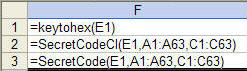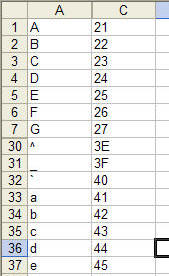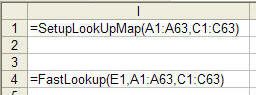You are on the Home/Excel/VBA/Simple Encoding pageShare Your

# Simple Encoding

This page contains four examples of how to use rather simple techniques to encode data.  Each of the techniques relies on a character-by-character encoding.  Consequently, none of them are useful for anything but trivial use.  In addition, two (#2 and #3) rely on Excel specific capability.

Common setup

Hex codes

Case insensitive encoding

Case sensitive encoding

Fast encoding

#### Common Setup

For all but the first example, the data are assumed to be organized as follows.

Column A of the active worksheet lists the character to be encoded.

Column C lists the corresponding code to use as a 2 character hex code.

The string being encoded is in E1.

The first three functions are used in an Excel worksheet as shown in Figure 2.Figure 2

The last function is demonstrated in Figure 3.Figure 1

#### Hex codes

The function KeyToHex simply converts the string in E1 into its hex equivalent.

```Public Function KeyToHex(ByVal sKey As String) As String
Dim i As Long, CodedKey As String
CodedKey = ""
For i = 1 To Len(sKey)
CodedKey = CodedKey & Right("0" & Hex(Asc(Mid(sKey, i, 1))), 2)
Next i
KeyToHex = CodedKey
End Function```

#### Case Insensitive Encoding

This technique leverages the fact that Excel VBA can use certain Excel functions.  Since the mapping information is in two ranges, the code uses the INDEX and MATCH functions to lookup the the code values corresponding to each character.

```Function SecretCodeCI(x As String, LookupVals, MatchingCodes) As String
'LookupVals is a Mx1 range, with each cell containing a single character _
MatchingCodes is a Mx1 range.
Dim i As Integer, Rslt As String
On Error GoTo ErrXIT
For i = 1 To Len(x)
Rslt = Rslt _
& MatchingCodes(Application.WorksheetFunction.Match(Mid(x, i, 1), LookupVals, 0))
Next i
SecretCodeCI = Rslt
Exit Function
ErrXIT:
SecretCodeCI = "Error: " & Err.Description & " (" & Err.Number & ")"
End Function
Sub testItCI()
Dim x, y
With ActiveSheet
Set x = .Range(.Cells(1, 1), .Cells(1, 1).End(xlDown))
Set y = x.Offset(, 2)
End With
MsgBox SecretCodeCI("nickelson", x, y)
End Sub```

#### Case Sensitive Encoding

Since the lookup functions in Excel (MATCH, VLOOKUP, etc.) are case insensitive, the code below uses the one Excel function that is case sensitive -- FIND -- to search a string.  Obviously, that requires that the mapping information be converted to a string.  The code uses the Excel TRANSPOSE and the VBA 6 Join functions to accomplish that.

Note that a variant that combines a component of the previous technique would be for the code to use the result of the FIND to index into the MatchingCodes range as above.  That would lift the restriction that each cell in MatchingCodes strictly contain a two character hex code.

```Function SecretCode(x As String, LookupVals, MatchingCodes) As String
'LookupVals is a Mx1 range, with each cell containing a single character _
MatchingCodes is a Mx1 range, with each cell containing a 2 character _
hex code.
Dim i As Integer, Rslt As String, sLookup, sMatches
'On Error GoTo ErrXIT
With Application.WorksheetFunction
sLookup = Join(.Transpose(LookupVals.Value), "")
sMatches = Join(.Transpose(MatchingCodes.Value), "")
End With
For i = 1 To Len(x)
Rslt = Rslt _
& Mid(sMatches, _
(Application.WorksheetFunction.Find(Mid(x, i, 1), sLookup) - 1) * 2 + 1, 2)
Next i
SecretCode = Rslt
Exit Function
ErrXIT:
SecretCode = "Error: " & Err.Description & " (" & Err.Number & ")"
End Function
Sub testIt()
Dim x, y
With ActiveSheet
Set x = .Range(.Cells(1, 1), .Cells(1, 1).End(xlDown))
Set y = x.Offset(, 2)
End With
MsgBox SecretCode("nickelson", x, y)
End Sub```

#### Fast Encoding

This technique initializes a data structure to facilitate a direct lookup of the the character being encoded.  It does this by putting the code corresponding to a particular character into an array location given by the ASCII code of the character.  For example, consider the character A.  Its ASCII code is 41.  Hence the array element 41 contains the code corresponding to the letter A.  Consequently, to find the code corresponding to A, all one needs to do is array(ASC("A"))

This technique bends Excel's rules of how a function is supposed to work.  The SetupLookupMap function actually initializes a global table that is used by the FastLookup function for the actual lookup.  To ensure that Excel calls the LookupMap function both when the string to be encoded changes and when the encoding map changes, the encoding map is passed to the function in the 2nd and 3rd arguments; however, the LookupVals function really uses only first argument.  This set of functions is used as shown below.Figure 3

`Option Explicit`
```Dim LookUpMap(0 To 255)
Function SetupLookUpMap(LookupVals, MatchingCodes)
'LookupVals and MatchingCodes must contain just one area _
The code currently doesn't cater to anything but a range
Dim i As Integer
For i = 1 To LookupVals.Cells.Count
LookUpMap(Asc(LookupVals.Cells(i).Value)) = MatchingCodes.Cells(i).Value
Next i
SetupLookUpMap = 0
End Function
Function FastLookup(x As String, LookupVals, MatchingCodes)
Dim i As Integer, Rslt As String
For i = 1 To Len(x)
Rslt = Rslt & LookUpMap(Asc(Mid(x, i, 1)))
Next i
FastLookup = Rslt
End Function```